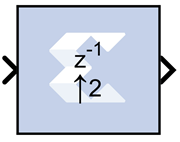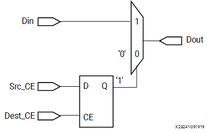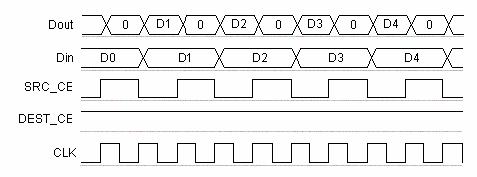# Up Sample - 2020.2 English

## Vivado Design Suite Reference Guide: Model-Based DSP Design Using System Generator (UG958)

Document ID
UG958
Release Date
2020-11-18
Version
2020.2 English

This block is listed in the following Xilinx® Blockset libraries: Basic Elements, and Index.

The Xilinx Up Sample block increases the sample rate at the point where the block is placed in your design. The output sample period is l/n, where l is the input sample period, and n is the sampling rate.The input signal is up sampled so that within an input sample frame, an input sample is either presented at the output n times if samples are copied, or presented once with (n-1) zeroes interspersed if zero padding is used.

In hardware, the Up Sample block has two possible implementations. If the Copy Samples option is selected on the block parameters dialog box, the Din port is connected directly to Dout and no hardware is expended. Alternatively, if zero padding is selected, a mux is used to switch between the input sample and inserted zeros. The corresponding circuit for the zero padding Up Sample block is shown below.

Figure 1. Zero Padding Up Sample Circuit## Block Interface

The Up Sample block receives two clock enable signals, Src_CE and Dest_CE. Src_CE is the clock enable signal corresponding to the input data stream rate. Dest_CE is the faster clock enable, corresponding to the output data stream rate. Notice that the circuit uses a single flip-flop in addition to the mux. The flip-flop is used to adjust the timing of Src_CE, so that the mux switches to the data input sample at the start of the input sample period, and switches to the constant zero after the first input sample. It is important to notice that the circuit has a combinational path from Din to Dout. As a result, an Up Sample block configured to zero pad should be followed by a register whenever possible.

Figure 2. Up Sample Output## Block Parameters

The block parameters dialog box can be invoked by double-clicking the icon in your Simulink® model.

Basic tab
Parameters specific to the Basic tab are as follows.
Sampling rate (number of output samples per input sample)
Must be an integer with a value of 2 or greater. This is the ratio of the output sample period to the input, and is essentially a sample rate multiplier. For example, a ratio of 2 indicates a doubling of the input sample rate. If a non-integer ratio is desired, the Up Sample block can be used in combination with the Down Sample block.
Copy samples (otherwise zeros are inserted)
Allows you to choose what to do with the additional samples produced by the increased clock rate. By selecting Copy Samples, the same sample is duplicated (copied) during the extra sample times. If this checkbox is not selected, the additional samples are zero.
Provide enable port
When checked, this option adds an en (enable) input port, if the Latency is specified as a positive integer greater than zero.
Latency
This defines the number of sample periods by which the block's output is delayed. One sample period can correspond to multiple clock cycles in the corresponding FPGA implementation (for example, when the hardware is over-clocked with respect to the Simulink® model). The user defined sample latency is handled in the Upsample block by placing shift registers that are clock enabled at the input sample rate, on the input of the block. The behavior of an Upsample block with non-zero latency is similar to putting a delay block, with equivalent latency, at the input of an Upsample block with zero latency.

Parameters used by this block are explained in the topic Common Options in Block Parameter Dialog Boxes.